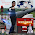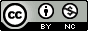## Monday, January 10, 2011

### R function for extracting F-test P-value from linear model object

I thought it would be trivial to extract the p-value on the F-test of a linear regression model (testing the null hypothesis R²=0). If I fit the linear model: fit<-lm(y~x1+x2), I can't seem to find it in names(fit) or summary(fit). But summary(fit)\$fstatistic does give you the F statistic, and both degrees of freedom, so I wrote this function to quickly pull out the p-value from this F-test on a lm object, and added it to my R profile. If there's a built-in R function to do this, please comment!

#### 6 comments:

1.That's the way you need to do it. In fact, that's how it is calculated in the source code to print.summary.lm(x,...):

pf(x\$fstatistic[1L], x\$fstatistic[2L], x\$fstatistic[3L],lower.tail = FALSE)

2.I could be totally wrong but I thing that

coef(summary(modelobject))["termname", "Pr(>|t|)"]

should pull out only the pvalue of the model.
Sorry if this isn't what you are looking for.

3.exactly what i was looking for! thanks :)

4.This is great. Thanks

5.What about the cor.test function?
> x <- c(44.4, 45.9, 41.9, 53.3, 44.7, 44.1, 50.7, 45.2, 60.1)
> y <- c( 2.6, 3.1, 2.5, 5.0, 3.6, 4.0, 5.2, 2.8, 3.8)

> lmp(lm(x~y))
 0.1081731
> cor.test(x,y)\$p.value
 0.1081731

6.thanks for sharing

Note: Only a member of this blog may post a comment.Getting Genetics Done by Stephen Turner is licensed under a Creative Commons Attribution-NonCommercial 3.0 Unported License.